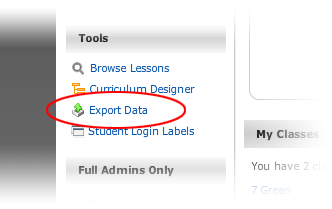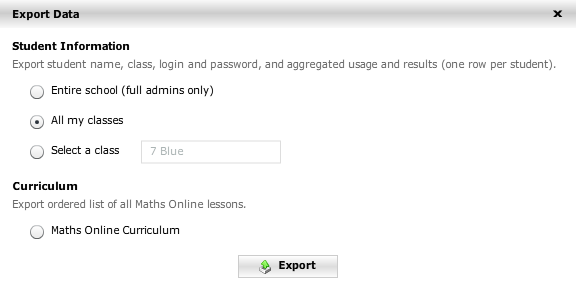Teacher’s User Guide

# Exporting data

To download data from the MathsBuddy system, from the Teacher Admin home page select the “Export Data” link shown below.The following pop-up appears.When any of the Student Information options are chosen, the following student data is retrieved from MathsBuddy:

• Class name
• Class group name
• First name
• Last name
• Login
• Password
• Number of logins
• Last login date and time
• Number of lessons attempted
• Number of lessons passed
• Average efficiency rating
• Number of tasks set for student
• Number of tasks completed on time
• Number of tasks completed late
• Number of certificates the student has attained
• Whether a parent of the student subscribes to the weekly report

When the Curriculum option is chosen, the complete menu structure of the MathsBuddy curriculum is exported. The following fields are included for each lesson in the curriculum:

• Unique lesson number
• Lesson name
• The course it is found in
• The stream it is found in
• The topic it is found in

All data is exported as CSV (comma separated values). By saving the downloaded file with a .csv extension, the file will automatically open in Excel, where the data can then be sorted, styled, printed and imported to other applications as required.

# Exporting data

To download data from the MathsBuddy system, from the Teacher Admin home page select the “Export Data” link shown below.The following pop-up appears.When any of the Student Information options are chosen, the following student data is retrieved from MathsBuddy:

• Class name
• Class group name
• First name
• Last name
• Login
• Password
• Number of logins
• Last login date and time
• Number of lessons attempted
• Number of lessons passed
• Average efficiency rating
• Number of tasks set for student
• Number of tasks completed on time
• Number of tasks completed late
• Number of certificates the student has attained
• Whether a parent of the student subscribes to the weekly report

When the Curriculum option is chosen, the complete menu structure of the MathsBuddy curriculum is exported. The following fields are included for each lesson in the curriculum:

• Unique lesson number
• Lesson name
• The course it is found in
• The stream it is found in
• The topic it is found in

All data is exported as CSV (comma separated values). By saving the downloaded file with a .csv extension, the file will automatically open in Excel, where the data can then be sorted, styled, printed and imported to other applications as required.